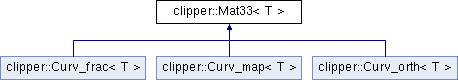Clipper
clipper::Mat33< T > Class Template Reference

3x3-matrix class More...

`#include <clipper_types.h>`

Inheritance diagram for clipper::Mat33< T >:List of all members.

## Public Member Functions

Mat33 ()
null constructor
Mat33 (const T &m00, const T &m01, const T &m02, const T &m10, const T &m11, const T &m12, const T &m20, const T &m21, const T &m22)
constructor
template<class TT >
Mat33 (const Mat33< TT > &m)
constructor: copy/convert
template<class TT >
Mat33 (const Mat33sym< TT > &m)
constructor: copy/convert from symmetric matrix
det () const
determinant
Mat33< T > inverse () const
inverse
Mat33< T > transpose () const
transpose
bool equals (const Mat33< T > &m, const T &tol) const
test equality
const T & operator() (const int &i, const int &j) const
get element
T & operator() (const int &i, const int &j)
set element
String format () const
return formatted String representation
bool is_null () const
test for null matrix (only valid for floating point types)

## Static Public Member Functions

static Mat33< T > identity ()
return identity matrix
static Mat33< T > null ()
return null matrix (only valid for floating point types)

## Friends

Vec3< T > operator* (const Mat33< T > &m, const Vec3< T > &v)
Matrix-vector product.
Vec3< T > operator* (const Vec3< T > &v, const Mat33< T > &m)
Vector-matrix product.
Mat33< T > operator* (const Mat33< T > &m1, const Mat33< T > &m2)
Matrix-matrix product.
Mat33< T > operator+ (const Mat33< T > &m1, const Mat33< T > &m2)
Matrix sum.
Mat33< T > operator- (const Mat33< T > &m)
Unary minus.

3x3-matrix class

## Friends And Related Function Documentation

template<class T = ftype>
 Vec3 operator* ( const Mat33< T > & m, const Vec3< T > & v ) ` [friend]`

Matrix-vector product.

Assumes a column vector

template<class T = ftype>
 Vec3 operator* ( const Vec3< T > & v, const Mat33< T > & m ) ` [friend]`

Vector-matrix product.

Assumes a row vector, i.e. equivalent to the matrix-vector product with the matrix trasposed

The documentation for this class was generated from the following file: